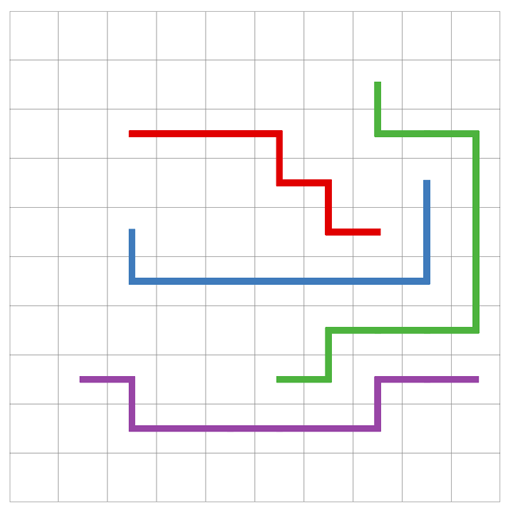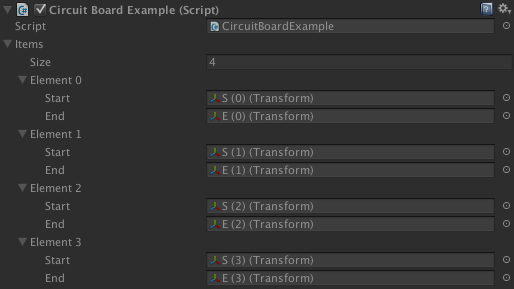# Example CircuitBoardExample.cs

This example shows how to use the Pathfinding.ITraversalProvider interface to generate paths like on a circuit-board.

This example shows how to use the Pathfinding.ITraversalProvider interface to generate paths like on a circuit-board.I.e paths that do not share any node. The image below shows a test case when using the script to calculate 4 paths on a small grid. The visualization of the paths has been improved manually using an external photo-editing application.The code has intentionally been left simple, so very little error checking and special case handling is done.

Note that finding paths on a circuit-board in an optimal way is a very hard problem (NP-Complete). For further information about that, see https://en.wikipedia.org/wiki/Multi-commodity_flow_problem.

Attach this script to any GameObject and fill in the 'items' array with the start and endpoints of each path.### See

Pathfinding.ITraversalProvider

`using UnityEngine;using System.Collections.Generic;using Pathfinding;[HelpURL("http://arongranberg.com/astar/documentation/stable/class_circuit_board_example.php")]public class CircuitBoardExample : MonoBehaviour { [System.Serializable] public class Item { public Transform start; public Transform end; } public Item[] items; class Blocker : ITraversalProvider { public HashSet<GraphNode> blockedNodes = new HashSet<GraphNode>(); public bool CanTraverse (Path path, GraphNode node) { // Override the default logic of which nodes can be traversed return DefaultITraversalProvider.CanTraverse(path, node) && !blockedNodes.Contains(node); } public uint GetTraversalCost (Path path, GraphNode node) { // Use the default costs return DefaultITraversalProvider.GetTraversalCost(path, node); } } void Update () { var traversalProvider = new Blocker(); // Calculate all paths in sequence. // It is important that they are not calculated in parallel // as we need to make sure that the paths do not visit the same nodes. // The result may vary significantly depending on the order in which // the paths are calculated. for (int index = 0; index < items.Length; index++) { var item = items[index]; // Create new path object ABPath path = ABPath.Construct(item.start.position, item.end.position); path.traversalProvider = traversalProvider; // Start calculating the path and put the path at the front of the queue AstarPath.StartPath(path, true); // Calculate the path immediately path.BlockUntilCalculated(); // Make sure the remaining paths do not use the same nodes as this one foreach (var node in path.path) { traversalProvider.blockedNodes.Add(node); } // Draw the path in the scene view Color color = AstarMath.IntToColor(index, 0.5f); for (int i = 0; i < path.vectorPath.Count - 1; i++) { Debug.DrawLine(path.vectorPath[i], path.vectorPath[i+1], color); } } }}`